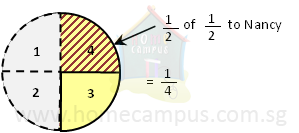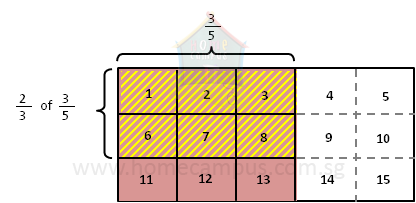## How to Multiply Fractions?

Practice Unlimited Questions

#### 2. Millie had   1 2   a pizza. She gave   1 2   of it to Nancy. What fraction of the pizza did Nancy receive?

Millie  =>
 1 2

Nancy  =>
 1 2
of
 1 21 2
of
 1 2

 1 2
×
 1 2

 1 x 1 2 x 2

 1 4

 1 4
of the pizza.
To find the product of fractions, multiply the numerators with each other and multiply the denominators with each other.

#### 3. What is 2 3 of 3 5 ?2 3
of
 3 5

 2 13
×
 31 5
(simplify)
 2 x 1 1 x 5

 2 5

 2 3
of
 3 5
is
 2 5
.

#### 4. What is 10 3 of 6 5 ?

 10 3
of
 6 5

 102 13
×
 62 15
(simplify)
 2 x 2 1 x 1

 4 1

=  4

 10 3
of
 6 5
is 4.